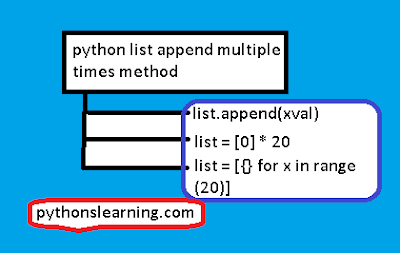# python list append multiple times :

Hello everyone, Today we are going to see How python list append multiple times? so Let's start:
If you want to append an item to a list in multiple times, for effectively doing this:python list append multiple times

For Example:
```
METHOD #01

list = []
```
xval = 0

for i in range(100):

list.append(xval)
``````
METHOD #02)
For Different data types:
list =  * 20
# [0, 0, 0, 0, 0, ...] It's get result all zeros up to 20 times
Similarly
list = ['pythonslearning'] * 100
# ['pythonslearning', 'pythonslearning', 'pythonslearning', 'pythonslearning', ...]
METHOD #03)

## `list = [{} for x in range(20)]`

In the above method the second one is best method for constant values, like as int or string. but why? the answer is when we use the syntax like `<list>*<number>`only a shallow copy is does and if we use something like as syntax [{}]*100 then it end up with 100 references to the same dictionary and hence change one of them would change them all. and the result is int and string are immutable.
You can use the `extend()` methods of that list If you want to add to an existing lists.
Method #01) `list.extend()`
Method #02)  generator expression:
````#l.``extend(generate_value() for _ in range(n))````
``````
This will add a value `n` times. Note that this will evaluate `generate_value()` each time, side-stepping issues with mutable values that other answers may have
```
>>> [] * 4
```
[, , , 
>>> _.append(1)

[[5, 5], [5, 5], [5, 5], [5, 5], [5, 5]]
>>> _

>>> [ for _ in range(5)]
[, , , , ]

[[5, 5], , , , ]
>>> _.append(1)

>>> _
``````
When using the First method like multiplication method,we end up with a lists of n reference to the same lists.
When using second method like a generator expressions or lists comprehension,for this method a new lists is created for each sub-item, so each item is a different value in every time.

Some FAQ about python list append multiple times

### 01)What is the difference between append and extend in Python?

Append:
Argument is a single elements to the end of a lists append adds its and length of the lists itself will increase by one.
Extend:
Extend is a arguments adding to each elements to the lists and extending the list and length of the lists increase by many position.

#### 02) How do I store multiple values in a list Python?

There are many ways to store multiple values in list python:
#1 We use the append() methods for this append() function
#2 we use the insert() methods for the insert() values in a list.
#3 we use the extend() methods they add multiple items to a list.
Summary:
In this article we covered different topic like How to append an item to a list in multiple times, difference between append and extend in Python,store multiple values in a list Python. about this section if you have any query then comment.
Tags:
How to python list append multiple times, use the `extend()` methods,difference between append and extend in Python,store multiple values in a list Python.
If you want to learn python course for free then click on python tutorials point and if you like this post then please comment and share
Best of luck!!

Previous
Next Post »

### comparison python vs r vs sas vs spss

python  vs r vs sas vs spss : Welcome everyone, Today we will see comparison between  r vs python vs sas vs spss . So Let's start : ...